# Hydrodynamic Dispersion of Solute under Homogeneous and Heterogeneous Reactions

Hydrodynamic Dispersion of Solute under Homogeneous and Heterogeneous Reactions
Department of Mathematics, National Institute of Technology Agartala, Tripura 799046, India

Corresponding Author Email:
apusaha_nita@yahoo.co.in
Page:
387-397
|
DOI:
https://doi.org/10.18280/ijht.370203
28 July 2017
|
Accepted:
7 May 2018
|
Published:
30 June 2019
| Citation

OPEN ACCESS

Abstract:

The present investigation deals with Taylor dispersion of reactive species in Casson liquid in an oscillatory flow because of the pulsatile pressure gradient. The solute is considered to be chemically active at the boundary and also participate a first order reaction within the bulk flow. To evaluate transport coefficients, Aris-Barton moment technique is considered. The solute transport process is discoursed in detailed with respect to yield stress, chemical reaction parameter, Womersly number etc. The study reveals that both wall absorption and bulk flow reaction have a significant response on dispersion phenomena. Both the chemical reactions agree to diminish the negative exchange coefficient and the apparent dispersion coefficient, however, increases the negative convection coefficient. The negative exchange coefficient is independent of yield stress but a significant variation is observed due to yield stress in the cases of negative convection coefficient and the apparent dispersion coefficient. The axial distribution of mean concentration is approximated by using the Hermite polynomial representation of central moments as a function of reaction rate parameters, wall absorbing parameter, yield stress etc. The present article may be useful for the studies related to physiological blood flow analysis.

Keywords:

Taylor dispersion, wall absorbtion, bulk flow reaction

1. Introduction

Taylor dispersion is the process of the transportation of a cloud contaminant by means of two independent mechanisms, convection and molecular diffusion. The Taylor dispersion is extensively connected to chemical engineering [1, 2], environmental protection [3–7], and physiological environment. The conventional endeavor of this sort of examination was started by Taylor . Subsequently, Taylor dispersion is generalized by Aris  by fixing the attention on the movement of the center of gravity of the slug and growth of its higher order moments. Barton  pointed out the mistake in the Aris solution methodology and resolved it accordingly. However, the solution acquired by Aris is satisfactory as it concerned the asymptotic behavior of the second moment. Another self-ruling technique is displayed by Gill & Sankarasubramanian , which is extended by more general inlet and boundary conditions in their successive papers [12–14].

There are a substantial number of reviews on Taylor dispersion that consider two independent perspectives in the Newtonian framework: (a) flow oscillation, and (b) chemical reaction. Both the viewpoints have their own particular significance while studying the dispersion process. Flow oscillation commonly appears in blood flow, airway gas mixing, extraction columns, etc. Scattering of species in the oscillatory stream is examined by Purtell , Ng , and Mazumder & Paul  etc. In the meantime, chemical reaction at the wall and within the bulk flow receives parallel attention. A variety of useful issues of mass transport involves wall reaction such as, open tube chromatography, environmental contaminant transport, and physiological transport etc. Again, the reaction at the bulk of the liquid is found in the hydrolysis of ester, gas absorption in an agitated tank with a chemical reaction etc. Some established work in this regard may include, among others, Aris , Gupta & Gupta , Sankarsubramanian & Gill , Ng  and Mazumder & Paul  and so forth.

The study of dispersion through non-Newtonian fluids receives less attention regardless of its abundant applications in polymers, biochemical processing, and cardiovascular flow etc. More specifically, the investigation of scattering in Casson liquids has applications in physiological liquid flow, as blood behavior mostly characterized by Casson fluid  under a particular environment. An investigation of scattering in non-Newtonian liquid (Bingham, Casson, and Power Law Fluids), with a view to understanding the rheology of blood on the dispersion process, was conducted by Sharp and then by Dash et al. . The theory is extended by Nagarani et al.  incorporating wall absorption through a pipe, and again, it has extended to the annular pipe in a subsequent paper . The authors  likewise concentrated the dispersion phenomena in Casson liquid through a conduit by applying the generalized dispersion model and establishing the functional relationship of a dispersion coefficient with a Womersley frequency number, Schmidt number, yield stress, and fluctuating pressure component. As of late, Rana & Murthy [25, 26] investigated the large time behavior of the axial dispersion process in an oscillatory flow in presence of wall reaction.

In literature, the studies on the combined effect of homogeneous and heterogeneous reactions on dispersion have been considered only in a Newtonian fluid. To the best of our knowledge, there is no attempt to observe the dispersion of species under simultaneous chemical reactions in the bulk flow and the tube wall in the non-Newtonian fluid. The present work, attempts are made to examine dispersion process in Casson liquid through a tube in presence of both reactions. In short the present study probably the fresh attempt and a generalization of the work of Rana and Murthy . The specific objective of the current study: (1) to cultivate a mathematical model of Taylor dispersion in oscillatory flow field; (2) to illustrate and characterize the behavior of dispersion coefficient w.r.t chemically active solute; and (3) to study the break through curve in above background.

2. Mathematical Model

2.1 Momentum equation

A unidirectional, axial, fully-developed laminar flow of an incompressible liquid is considered through a circular pipe of radius $\hat{a}$. The coordinate system is chosen here is cylindrical, where the axial and radial co-ordinates are represented by $\hat{Z}$ and $\hat{r}$ (cap denotes dimensional quantity). Axi-symmetry are assumed and hence all quantities are independent of $\theta$ .

We assume the regions of Casson liquid and the plug flow in the Casson region by $\hat{\mathfrak{R}}_{c}$ and $\hat{\mathfrak{R}}_{p}$ respectively, where

$\hat{\mathfrak{R}}_{c}=\{(\hat{r}, \hat{z}) : 0 \leq \hat{r} \leq \hat{a},-\infty \leq \hat{z} \leq \infty\}$ (1)

$\hat{\mathfrak{R}}_{p}=\left\{(\hat{r}, \hat{z}) : 0 \leq \hat{r} \leq \hat{r}_{p},-\infty \leq \hat{z} \leq \infty\right\}$  (2)

The governing equation of motion for the flow in the axial direction are given by

$\hat{\rho} \frac{d \hat{u}}{d \hat{t}}=-\frac{d \hat{p}}{d \hat{z}}-\frac{1}{\hat{r}} \frac{\partial\left(\hat{r} \hat{\tau}_{\hat{z} \hat{r}}\right)}{\partial \hat{r}}$  (3)

where $\hat{\rho}$ is the density of the flowing liquid. The axial pressure gradient represented by $\frac{d \hat{p}}{d \hat{z}}=p_{0}+p_{1} \sin (\widehat{\omega} \hat{t})$, $p_{0}$, $p_{1}$ are the steady and oscillatory part of the pressure gradient and $\widehat{\omega}$ is the frequency of the pressure fluctuation.

Constitutive equation

$\left. \begin{array}{*{35}{l}} \hat{\tau }_{\hat{z}\hat{r}}^{\frac{1}{2}}={{{\hat{\tau }}}^{\frac{1}{2}}}+{{\left( -{{\mu }_{\infty }}\frac{\partial \hat{u}}{\partial \hat{r}} \right)}^{\frac{1}{2}}}\ ,\quad {{{\hat{\tau }}}_{\hat{z}\hat{r}}}\ge {{{\hat{\tau }}}_{{\hat{y}}}} \\ \frac{\partial \hat{u}}{\partial \hat{r}}=0\ ,\quad \text{ }{{{\hat{\tau }}}_{\hat{z}\hat{r}}}\le {{{\hat{\tau }}}_{{\hat{y}}}}\text{ } \\ \end{array} \right\}$  (4)

where $\hat{\tau}_{\hat{y}}$ is the yield stress and $\hat{\mu}_{\infty}$ the Newtonian viscosity of the liquid. From the Eq. (4), it is obvious that whenever $\hat{\tau}_{\hat{z} \hat{r}} \leq \hat{\tau}_{\hat{y}}$, plug flow will occur.

The boundary conditions are

$\begin{array}{ll}{\hat{\tau}_{\hat{z} \hat{r}} \text { is finite }} & {\text { at } \hat{r}=0} \\ {\hat{u}=0} & {\text { at } \hat{r}=\hat{a}}\end{array} \}$   (5)

The following dimensionless quantities are used

\left. \begin{align} & t=\frac{\hat{D}\ \hat{t}}{{{{\hat{a}}}^{2}}},\quad r=\frac{{\hat{r}}}{{\hat{a}}},\quad z=\frac{\widehat{D}\hat{z}}{{{{\hat{a}}}^{2}}{{u}_{0}}},\quad C=\frac{{\hat{C}}}{{{C}_{0}}},\quad u=\frac{{\hat{u}}}{{{u}_{0}}},\quad \\ & {{u}_{0}}=-\frac{{{{\hat{a}}}^{2}}}{4{{\mu }_{\infty }}}{{p}_{0}},\quad {{\tau }_{zr}}=\frac{{{{\hat{\tau }}}_{\hat{z}\hat{r}}}}{{{\mu }_{\infty }}(\frac{{{u}_{0}}}{{\hat{a}}})},\quad {{\tau }_{y}}=\frac{{{{\hat{\tau }}}_{{\hat{y}}}}}{{{\mu }_{\infty }}(\frac{{{u}_{0}}}{{\hat{a}}})},\text{ }e=\frac{{{p}_{1}}}{{{p}_{0}}} \\ \end{align} \right\} (6)

Using the scaling as given in Eq. (6), the initial-boundary value problem (IBVP) i.e., Eqns. (3)-(5) reduces to.

$\frac{1}{Sc}\frac{\partial u}{\partial t}=4p(t)-\frac{1}{r}\frac{\partial }{\partial r}\left( r{{\tau }_{rz}} \right)$    (7)

$\left. \begin{array}{*{35}{l}} \tau _{_{zr}}^{\frac{1}{2}}={{\tau }^{^{\frac{1}{2}}}}+{{\left( -\frac{\partial u}{\partial r} \right)}^{\frac{1}{2}}}\quad {{\tau }_{_{zr}}}\ge {{\tau }_{y}}, \\ \frac{\partial u}{\partial r}=0\quad \text{ }{{\tau }_{_{zr}}}\le {{\tau }_{y}},\text{ } \\ \end{array} \right\}$  (8)

$\left. \begin{array}{*{35}{l}} {{\tau }_{yz}}\ \text{is finite}\quad \text{at}\ r=0 \\ u=0\quad \qquad \text{at}\ r=1 \\ \end{array} \right\}$  (9)

2.2 Transport Equation

In the uni-directional flow described above, a reactive solute with initial concentration $\hat{C}(0, \hat{r}, \hat{z})$ is introduced. The dimensionless convection-diffusion equation satisfied by the solute concentration is

$\frac{\partial C}{\partial t}+\ u(r,t)\frac{\partial C}{\partial z}=\frac{1}{r}\frac{\partial }{\partial r}\left( r\frac{\partial C}{\partial r} \right)+\frac{1}{P{{e}^{2}}}\frac{{{\partial }^{2}}C}{\partial {{z}^{2}}}-\kappa C$ (10)

$C(0,r,z)=\delta (z),\quad (0<r<1)$   (11)

$\frac{\partial C}{\partial r}\text{ }=0\qquad \text{at}\quad r=0$    (12)

$\frac{\partial C}{\partial r}=-\beta C\quad \text{at}\quad r=1$ (13)

$C(t,r,\infty )=0=\frac{\partial C}{\partial z}(t,r,\infty )$  (14)

Here $\kappa\left(=\frac{\widehat{\kappa} \hat{a}^{2}}{\widehat{D}}\right)$ is the bulk flow reaction rate, $\beta(=\hat{\beta} \widehat{a})$ is wall absorption and $P e\left(=\frac{u_{0} \hat{a}}{\widehat{D}}\right)$ is the Péclet number that measures the ratio of the characteristic time of the diffusion process to that of the convection process. The Eq. (13) tells that the wall of the conduct is permeable whereas Eq. (14) reflects the fact that the total amount of solute is finite.

3. Solution Methodology

3.1 Velocity distribution

To solve the coupled Eqns. (7) & (9), we consider regular perturbation method by treating 1$/ S c$ as a perturbation parameter, as. $S c$ is very large in case of blood flow. The velocity distribution  is:

$u(r,t)=\left\{ \begin{array}{*{35}{l}} {{u}^{-}}(r,t),\text{ }r\in {{\Re }_{p}} \\ {{u}^{+}}(r,t),\text{ }r\in {{\Re }_{c}}-{{\Re }_{p}} \\ \end{array} \right.$  (15)

where

${{u}^{-}}(r,t)=\frac{p(t)}{2}\left[ 1-\frac{8}{3}\sqrt{{{r}_{p}}}+2{{r}_{p}}-\frac{1}{3}r_{_{p}}^{2} \right]$$\text{ }-\epsilon \frac{{p}'(t)}{32}\left[ 3-\frac{1147}{147}\sqrt{{{r}_{p}}}+\frac{320}{63}{{r}_{p}} \right.$$ \left. \text{ }+\frac{4}{3}r_{_{p}}^{2}-\frac{16}{9}r_{_{p}}^{5/2}+\frac{65}{441}{{r}^{4}} \right]$ (16)

${{u}^{+}}(r,t)=p(t)[(1-{{r}^{2}})+2{{r}_{p}}(1-r)-\frac{8}{3}\sqrt{{{r}_{p}}}(1-{{r}^{3/2}})] $$\text{ }-\epsilon \frac{{p}'(t)}{2}\left[ \frac{3}{16}-\frac{{{r}^{2}}}{16}(4-{{r}^{2}})-\frac{r_{p}^{2}}{16}\left( \frac{1144}{147} \right. \right.$$ \left. \left. \text{ }-\frac{16}{3}(r+{{r}^{3/2}})+\frac{424}{147}{{r}^{7/2}} \right) \right] $$\text{ }-\epsilon \frac{{p}'(t)}{32}{{r}_{p}}\left( \frac{320}{63}+\frac{128}{63}{{r}^{3/2}}-\frac{64}{9}{{r}^{3/2}} \right) (17) where u^{-}(r, t) is the velocity in the plug flow region \mathfrak{R}_{p} having plug radius r_{p}=\frac{\tau_{y}}{p(t)}, \left(p(t)=1+e \sin \left(\alpha^{2} S c t\right)\right., \alpha^{2}=\frac{\widehat{\omega} \hat{a}^{2}}{v}, \alpha is Womersely frequency parameter, e is the amplitude of the fluctuating pressure component) and u^{+}(r, t) is the velocity of the remaining portion \left(\Re_{p}-\Re_{c}\right) of the tube. 3.2 Aris-Barton approach Following the method of integral moment proposed by Aris  and modified by Barton , we define the p-th moment of the distribution of the solute in the filament through r at time t as, ${{C}_{p}}\left( t,r \right)=\underset{-\infty }{\overset{+\infty }{\mathop \int }}\,{{z}^{p}}C\left( t,r,z \right)dz$ (18) and the concentration distribution of the solute over the cross-section of the tube is given by, ${{\bar{C}}_{p}}\left( t \right)=\frac{\int\limits_{0}^{2\pi }{d\theta }\mathop{\int }_{0}^{1}r{{C}_{p}}\left( t,r \right)dr}{\mathop{\int }_{0}^{2\pi }d\theta \mathop{\int }_{0}^{1}rdr}$ (19) So using Eq. (18), the diffusion Eq. (10) subject to initial and boundary conditions (11)-(13) can be rewritten as: \frac{\partial {{C}_{p}}}{\partial t}-\frac{1}{r}\frac{\partial }{\partial r}\left( r\frac{\partial {{C}_{p}}}{\partial r} \right)=-pu\left( r \right){{C}_{p-1}}$$ \text{ }~\text{ }+\frac{1}{P{{e}^{2}}}p\left( p-1 \right){{C}_{p-2}}-\kappa {{C}_{p}}$  (20)

With

$C_{p}(0, r)=\left\{\begin{array}{cl}{\frac{1}{P e^{2}}} & {\text { for } p=0} \\ {0} & {\text { for } p>0}\end{array}\right.$

$\frac{\partial C_{p}}{\partial r}=\left\{\begin{array}{c}{0 \quad \text { at } r=0} \\ {-\beta C_{p} \text { at } r=1}\end{array}\right.$   (21)

Eq. (20) subject to initial and boundary condition (21) have been solved numerically by applying finite difference method based on Crank-Nicholson implicit scheme. At the beginning, discretized the Eq. (20) and Eq. (21) in the domain (i.e. $0\le r\le 1$ ). As a result the differential equation converted into following difference equation at each grid point $(i,j)$ .

${{P}_{j}}{{C}_{p}}(i+1,j+1)+{{Q}_{j}}{{C}_{p}}(i+1,j)$$\text{ }+{{R}_{j}}{{C}_{p}}(i+1,j-1)={{S}_{j}}$  (22)

where ${{P}_{j}},{{Q}_{j}},{{R}_{j}}$ and ${{S}_{j}}$  are the matrix elements.

The finite difference form of the initial condition is,

$\left. \begin{matrix} {{C}_{p}}(1,j) & = & 1\quad \text{for}\quad p=0 \\ {} & = & 0\quad \text{for}\quad p\ge 0 \\ \end{matrix} \right\}\$  (23)

and that of boundary conditions are

$\begin{matrix} {{C}_{p}}(i+1,0) & = & {{C}_{p}}(i+1,2), \\ {{C}_{p}}(i+1,{{M}_{2}}+1) & = & {{C}_{p}}(i+1,{{M}_{2}}-1) \\ {} & {} & -2\beta \Delta r{{C}_{p}}(i+1,{{M}_{2}}). \\ \end{matrix}\$  (24)

The grid i indicating the time and j indicating spatial coordinate. Thus i=0 corresponds to the initial time and the subsequent time is obtained from the relation ${{t}_{i}}=\Delta t\times (i-1)$  where $\Delta t$  is the time increment. Now to discretize the spatial coordinate, we first determine the plug region with the help of the relation ${{r}_{p}}={{\tau }_{y}}/p(t)$ , accordingly, we have set two different step width $\Delta {{r}_{1}}$  and $\Delta {{r}_{2}}$ , first one for plug core region ${{\Re }_{p}}$  and other one for the reaming part $({{\Re }_{c}}-{{\Re }_{p}})$.

where

${{r}_{j}}=\left\{ \begin{array}{*{35}{l}} (j-1)\times \Delta {{r}_{1}}\qquad \text{ in}\quad {{\Re }_{p}} \\ {{r}_{p}}+(j-1)\times \Delta {{r}_{2}}\qquad \text{in}\quad {{\Re }_{c}}-{{\Re }_{p}} \\ \end{array} \right.$

Finally, the system of algebraic equations is solved by Thomas algorithm  with the view of prescribed initial and boundary conditions. All these computations are performed by devolving a Matlab code.

In our analysis, we choose $\Delta t=0.00001,\Delta {{r}_{1}}=\frac{{{r}_{p}}-0}{{{M}_{1}}-1}$ and $\Delta {{r}_{2}}=\frac{1-{{r}_{p}}}{{{M}_{2}}-1}$, where ${{M}_{1}}$ and ${{M}_{2}}$ are a number of grid point in the ${{\Re }_{p}}$ and $({{\Re }_{c}}-{{\Re }_{p}})$. In all the cases we have taken $Pe={{10}^{3}}$. Once the value of ${{C}_{P}}(i,j)$ is calculated, from Eq.(19) one can able to calculate ${{\bar{C}}_{p}}$ by applying any suitable method of numerical integration with the known values of $u(r,t)$ and ${{C}_{p}}$. In the present article, we follow Simpson 1/3 rule.

The p-th order central moment of the concentration distribution about the mean can be defined as

${{\mu }_{p}}(t)\ =\ \frac{\int\limits_{0}^{1}{\int\limits_{0}^{2\pi }{\underset{-\infty }{\overset{+\infty }{\mathop \int }}\,}}r{{(z-{{z}_{g}})}^{p}}C(t,r,z)drd\theta dz}{\int\limits_{0}^{1}{\int\limits_{0}^{2\pi }{\underset{-\infty }{\overset{\infty }{\mathop \int }}\,}}rC(t,r,z)drd\theta dz}\$(25)

where ${{z}_{g}}={{{{\bar{C}}}_{1}}}/{{{{\bar{C}}}_{0}}}\;$  is the centroid or first moment of the solute and $\overline{C}_{0}$ represents the total mass of the reactive solute in the whole volume of the tube. The expressions for central moments can be obtained from (25) as,

$\left. \begin{array}{*{35}{l}} {{\mu }_{0}}\left( t \right)=1 \\ {{\mu }_{1}}\left( t \right)=0 \\ {{\mu }_{2}}\left( t \right)=\frac{{{{\bar{C}}}_{2}}}{{{{\bar{C}}}_{0}}}-z_{g}^{2} \\ {{\mu }_{3}}\left( t \right)=\frac{{{{\bar{C}}}_{3}}}{{{{\bar{C}}}_{0}}}-3{{z}_{g}}{{\mu }_{2}}-z_{g}^{3} \\ {{\mu }_{4}}\left( t \right)=\frac{{{{\bar{C}}}_{4}}}{{{{\bar{C}}}_{0}}}-4{{z}_{g}}{{\mu }_{3}}-6z_{g}^{2}{{\mu }_{2}}-z_{g}^{4} \\ \end{array} \right\}$  (26)

4. Results and Discussion

The present analysis deals with the longitudinal dispersion of reactive solute in an oscillatory flow when it is introduced into a circular tube by modeling the flowing fluid as a Casson liquid. In the introduction section, we have underlined the significance of reactive solute in dispersion process and accordingly, we have modeled the problem. The effect of homogeneous and heterogeneous reaction on dispersion is highlighted against the foundation of the outcomes in regard to inert solute. The whole analysis is performed on the basis of Aris-Barton moment method and the higher order moment equations are solved numerically. Although the numerical scheme adopted in the present review is unconditionally stable, but we have validated our result by some existing work. The results are found to be in good agreement with sankarasubramanian & Gill  when $\tau_{y}=0$ , Nagarani et al.  when $e=0$ and Rana & Murty  when $\kappa=0$ . Before going to definite discourse, we have fixed the parameters involved in the analysis by considering previous investigation and rheological perspective. The table documented below are the conceivable range for the involving parameters:

Table 1. Ranges of controlling parameter considered in the present study

 Parameter Range of Value $\tau_{y}$ 0-0.05 $\kappa$ 0-20 $\alpha$ 0.1-4 $\beta$ 0-100 $e$ 0-0.5

In the present analysis, we mainly focus our attention in three transport coefficients viz. Exchange coefficient, Convection coefficient and dispersion coefficient. We also study the mean concentration on a similar foundation. All three transport coefficient and mean concentration are function of central moment defined in Eq. (26). The interrelated relationship of the four elements with central moment, and the parametric effect on these four element are elaborated in the following subsections:

4.1 Exchange coefficient

The exchange coefficient $K_{0}$ appears due to reaction and can be figured from the analogy that the total mass decays exponentially with time as per the following relation:

${{K}_{0}}(t)={{\bar{C}}_{0}}(0){{e}^{\int_{0}^{t}{{{K}_{0}}}(t)dt}}$  (27)

From Eq. (27) it is clear that $K_{0}$  is function of $\beta$ and $\boldsymbol{K}$ only. The variation of $K_{0}$ with time for different $\beta$ and $\boldsymbol{K}$ is portrayed in Figure $-K_{0}$ is an increasing function of time but an equilibrium gradually set up between solute transfer from the boundary to the central core region due to molecular diffusion and solute transfer from boundary into wall. Also $-K_{0}$ increases with the increment of $\beta$ , as an increase in $\beta$  consumes material more rapidly than it can be supplied by molecular diffusion, consequently, the system becomes diffusion controlled and reaches to its steady state for large $\beta$. In like manner, increase in $\boldsymbol{K}$ results increase in $-K_{0}$. The above perception furthermore maintained by Figure 2 and Figure 3. When $\boldsymbol{K}=0$ , the negative exchange curve w.r.t time qualitatively and quantitatively agree with sankarasubramanian & Gill , Nagarani et al.  and Rana & Murty .

4.2 Convection coefficient

Convection coefficient arises naturally in the study due to the fluid movement. As the center of mass moves with fluid velocity, the Convection coefficient $K_{1}$ is given by,

${{K}_{1}}=\frac{d{{z}_{g}}}{dt}$    (28)

Convection coefficient is the function of $\tau_{y}$, $e$, $\beta$ and $\boldsymbol{K}$. The first two parameters are due to fluid velocity and last two, because of the consideration that the species is reactive. The behavior of $-K_{1}$ is pulsatile in nature, initially, species is convected with the velocity of the fluid along the flow, hence the underlying speed of the species relies on $\tau_{y}$ [Figure 4(a)].The amplitude of fluctuation of $-K_{1}$ initially varies with time and reaches to the constant value in the developed time [Figure 4(a, b)].This non-transient state depends on other parameters also. By increasing $e$, one can also increase the amplitude $-K_{1}$ [Figure 5(a, b)]. As expected, increase in $\tau_{y}$ decreases the convection coefficient $-K_{1}$ [Figure 4(a, b)].

Figure 6(a, b) and Figure 7, uncovers the reliance of wall observation on negative convection coefficient $-K_{1}$ at a small and large time. From Figure 6(a, b) it is observed that with the increase in $\beta$ negative convection coefficient $-K_{1}$ increases, as the wall reaction enhance the transport of cloud contaminant towards the wall, subsequently $-K_{1}$ increases, with $\beta$. An identical behavior is observed for $\boldsymbol{K}$ [Figure 8 and Figure 9(a, b)].

## figure_1.png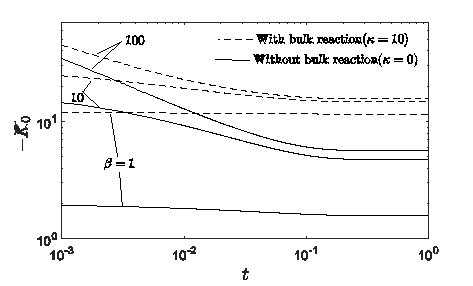Figure 1. Variation of negative exchange coefficient with time

## figure_2.png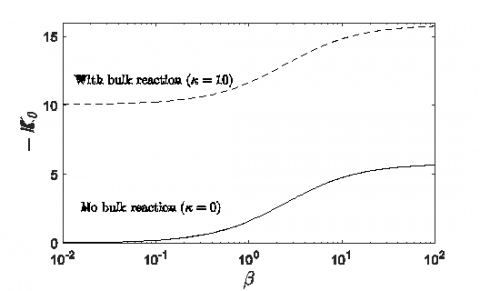Figure 2. Variation of negative exchange coefficient -K against β at t=1

## figure_3.pngFigure 3. Variation of negative exchange coefficient -K0 against k at t=1

4.3 Dispersion coefficient

Because of velocity shear, the axial diffusion is negligible compare to radial diffusion. Aris  showed that the rate of change of variance is proportional to the sum of molecular diffusion coefficient along the axial direction and apparent dispersion coefficient (Taylor dispersion coefficient). Since the axial diffusion is negligible compared to the lateral diffusion, the apparent dispersion coefficient $D_{e f f}$ can be written as

${{D}_{eff}}=\frac{1}{2}\frac{d{{\mu }_{2}}}{dt}\$   (29)

The apparent dispersion coefficient depends on yields stress $\tau_{y}$, the amplitude of pressure fluctuation $e$, wall absorption parameter $\beta$, womersly number $\alpha$ and bulk flow reaction parameter $\kappa$. As like negative convection coefficient, dispersion coefficient is also oscillatory in nature, and varying its amplitude initially, and gradually reaches to its non-transient state. As mentioned above this non-transient state depends on other factors like $\tau_{y}$, $e$, $\alpha$ etc. Figure 10(a, b) and Figure 11(a, b) attracted to underscore the impact of yield stress $\tau_{y}$ on dispersion coefficient and is seen that the apparent dispersion coefficient $D_{e f f}$ is decreasing with $\tau_{y}$ at a small as well as large time. The reason behind the decrement is velocity, the increase in yield stress, velocity decrease which, in turns decrease the dispersion coefficient. To observe the effect of wall absorption parameter, Figure 12 is presented and noticed that the wall absorption $\beta$ helps to diminish the effective dispersion coefficient $D_{e f f}$. Variation of asymptotic dispersion coefficient $D_{e f f}$ with respect to $\beta$ for different values of yield stress is also examined [Figure 16] at time t=1. The physical explanation for this effect is identical to that of Sankarasubramanian and Gill  Notable that a similar kind of trend is observed in the case of bulk flow reaction parameter in Figure 13 and Figure 17. This decrease in the dispersion coefficient with the increase in the reaction rate constant is based on the sound physical ground. The increase in $\kappa$  leads to the growth in the number of moles of solute undergoing chemical reaction resulting in a drop-in dispersion coefficient. It is worth noting that the value of dispersion become negative for the high value of Womersly number and amplitude of pressure pulsation [Figure 14(a, b) and Figure 15(b)], i.e. species move back with the flow, and the reason is quite natural as back flow appears in the motion due to pulse.

4.4 Mean concentration

The moment method no longer gives any immediate expression for mean concentration but it is conceivable to surmise the mean concentration which can also be achieved by means of Hermite polynomials for the representation of non-Gaussian curve [29, 30] with the aid of first four central moments as follows,

${{C}_{m}}(z,t)={{\bar{C}}_{0}}(t){{e}^{-{{\eta }^{2}}}}\sum\limits_{n=0}^{\infty }{{{a}_{n}}}(t){{H}_{n}}(\eta )\$  (30)

where $\eta=\frac{\left(z-z_{g}\right)}{\sqrt{2 \mu_{2}}}$, $z_{g}=\frac{\overline{C}_{1}}{\overline{c}_{0}}$ and $H_{i}$, the Hermite polynomials, satisfy the recurrence relation

${{H}_{i+1}}(\eta )=2\eta {{H}_{i}}(\eta )-2i{{H}_{i-1}}(\eta ),\text{ }\ \ i=0,1,2,\ldots$  (31)

in which $H_{0}(\eta)=1$

The coefficients $a_{i}$'s are

${{a}_{0}}=\frac{1}{\sqrt{2\pi {{\mu }_{2}}}},\text{ }{{a}_{1}}=0,\text{ }{{a}_{2}}=0,\text{ }{{a}_{3}}=\frac{\sqrt{2}{{a}_{0}}{{\nu }_{2}}}{24},\text{ }{{a}_{4}}=\frac{{{a}_{0}}{{\nu }_{3}}}{96}.$

## figure_4a.png## figure_4b.pngFigure 4. Variation of negative convection coefficient with time for different values of yield stress when $\beta=1$, $e=0.5$, $\alpha=0.5$, $\kappa=10$. (a) Small time (b) Large time

## figure_5a.png## figure_5b.pngFigure 5. Variation of negative convection coefficient with time for different values of amplitude of pressure fluctuation when $\beta=1$, $\tau_{y}=0.05$, $\alpha=0.5$, $\kappa=10$. (a) Small time (b) Large time

## figure_6a.png## figure_6b.pngFigure 6. Variation of dispersion coefficient with time for different values of absorption parameter when $\tau_{y}=0.05$, $e=0.5$, $\alpha=0.5$, $\kappa=10$. (a) Small time (b) Large time

## figure_7.pngFigure 7. Variation of negative convection coefficient $-K_{1}$ against $\beta$ when $\kappa=10$ and $\tau_{y}=0.02$ at $e=0$

## figure_8.pngFigure 8. Variation of negative convection coefficient against $\kappa$ when $\beta=1$  and $\tau_{y}=0.02$ at $e=0$

## figure_9a.png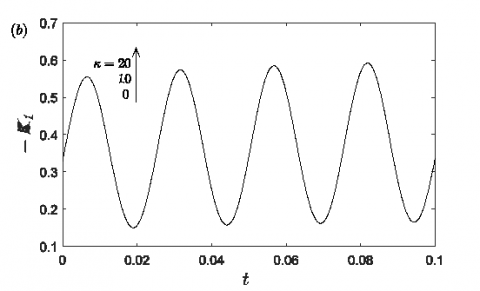## figure_9b.pngFigure 9. Variation of negative convection coefficient with time for different values of reaction parameter when $\beta=1$, $e=0.5$, $\alpha=0.5$, $\tau_{y}=0.05$. (a) Small time (b) Large time

## figure_10a.png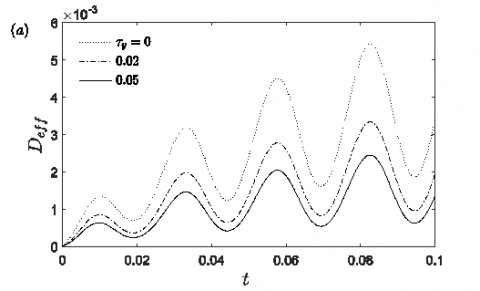## figure_10b.png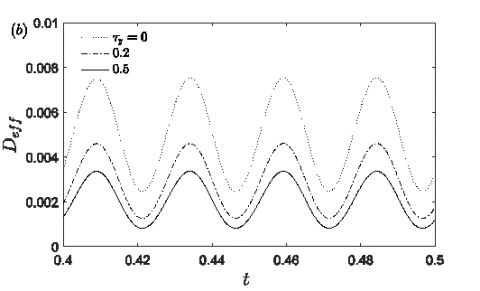Figure 10. Variation of negative Dispersion coefficient with time for different values of yield stress when $\beta=1$, $e=0.5$, $\alpha=0.5$, $\kappa=10$. (a) Small time (b) Large time

## figure_11a.png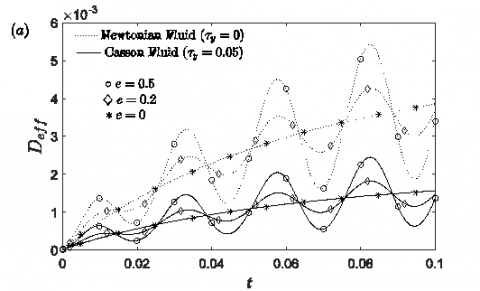## figure_11b.png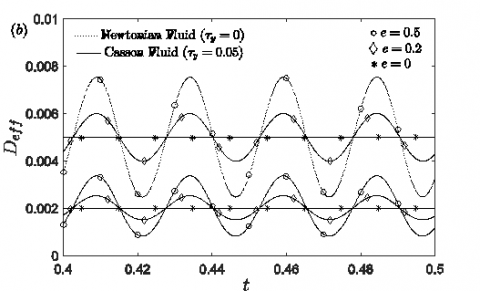Figure 11. Variation of Dispersion coefficient with time for different values of $e$ when $\beta=1$, $\kappa=10$, $\alpha=0.5$. (a) Small time (b) Large time

## figure_12a.png## figure_12b.pngFigure 12. Variation of dispersion coefficient with time for different values of absorption parameter when $\tau_{y}=0.05$, $e=0.5$, $\alpha=0.5$, $\kappa=10$ (a) Small time (b) Large time

## figure_13a.png## figure_13b.png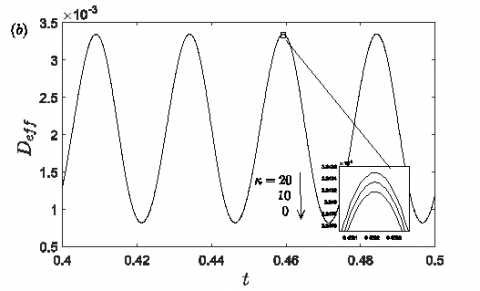Figure 13. Variation of Dispersion coefficient with time for different values of reaction parameter when $\tau_{y}=0.05$, $e=0.5$, $\alpha=0.5$, $\beta=1$. (a) Small time (b) Large time

## figure_14a.png## figure_14b.pngFigure 14. Variation of Dispersion coefficient with time for different values of absorption parameter when $\kappa=10$, $e=0.5$, $\alpha=0.5$, $\tau_{y}=0.05$. (a) Small time (b) Large time

## figure_15a.png## figure_15b.pngFigure 15. Variation of dispersion coefficient with time for when, $e=0.5$ , $\kappa=10$,  $\tau_{y}=0.05$. (a) $\beta=1$ (b)$\alpha=4$

## figure_16.png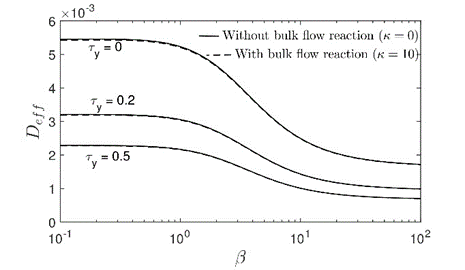Figure 16. Variation of Dispersion coefficient $D_{e f f}$ against $\beta$ at $t=1$ when $e=0.5$, $\alpha=0.5$.

## figure_17.pngFigure 17. Variation of Dispersion coefficient $D_{e f f}$ against $\kappa$ at $t=1$ when $\beta=1$, $e=0.5$, $\tau_{y}=0.05$

## figure_18a.png## figure_18b.png## figure_18c.png## figure_18d.png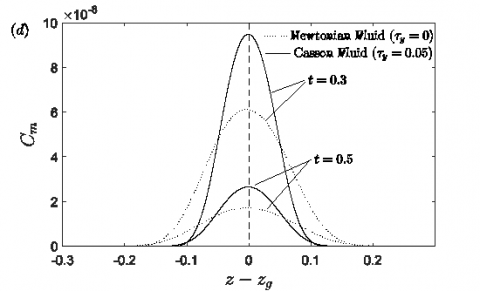Figure 18. Distribution of mean concentration against the axial distance for (a) different values of the absorption parameter when $\tau_{y}=0.05$, $\kappa=10$, $e=0.5$, $\alpha=0.5$. (b) different values of the reaction parameter when $\tau_{y}=0.05$, $\beta=1$, $e=0.5$, $\alpha=0.5$. (c) different values of yield stress when $\kappa=10$, $\beta=1$, $e=0.5$, $\alpha=0.5$. (d) different time when $\kappa=10$, $\beta=1$, $e=0.5$

5. Conclusion

In this work, longitudinal dispersion of pulsatile stream of reactive species in presence of homogeneous and heterogeneous reaction are examined. The flow pulsation is arisen due to a periodic pressure gradient. It is found that for homogeneous reaction in the bulk of the liquid, the apparent dispersion coefficient and negative exchange coefficient decrease with increase in the reaction rate constant, the effect similar to the irreversible reaction in the boundary. In any case, both the reaction enhances the negative convection coefficient. The negative convection coefficient and apparent dispersion coefficient function of wall absorbing parameter yield stress while exchange coefficient is independent of yield stress. It is found that yield stress is in favor of decrement of the negative convection coefficient and the apparent dispersion coefficient. The present work clarifies the impacts of pulsatility, first order homogeneous and heterogeneous chemical reaction in Casson liquid, considerably nearer to that in a physiological circumstance in contrast with literature, yet far away to merit use with regards to a clinical circumstance.

Acknowledgement

The authors greatly acknowledge valuable suggestions and comments by Dr. Suvadip Paul, Dept. of Mathematics, Government Degree College, Dharmanagar, Tripura, 799250 India.

Nomenclature
 α radius of the pipe $\tau_{y}$ yield stress $\mathcal{U}$ axial velocity $\mu_{\infty}$ Newtonian viscosity C solute concentration D molecular diffusivity $\boldsymbol{K}$ bulk flow reaction parameter $\delta$ dirac delta function $\beta$ absorption parameter $Pe$ Péclet number $S c$ Schmidt number Hi Hermite polynomials rp plug core radius Cp moment of the solute concentration $\mu_{p}$ p-th order central moment of the solute concentration $K_{0}$ exchange coefficient
References

 Harper, R.N., Boyce, C.M., Scott, S.A. (2013). Oxygen carrier dispersion in inert packed beds to improve performance in chemical looping combustion. Chemical Engineering Journal, 234: 464–474. https://doi.org/10.1016/j.cej.2013.08.090

 Kalman, S.S. (2015). Three plugs model. Powder Technology, 283: 579–592. https://doi.org/10.1016/j.powtec.2015.05.047

 Wang, P., Chen, G.Q. (2015). Environmental dispersion in a tidal wetland with sorption by vegetation. Communications in Nonlinear Science and Numerical Simulation, 22(1-3): 348–366. https://doi.org/10.1016/j.cnsns.2014.09.002

 Wang, P., Li, Z., Huai, W.X., Chen, B., Li, J.S., Hayat, T., Alsaedi, A., Chen, G.Q. (2014). Indicators for environmental dispersion in a three-layer wetland: Extension of Taylor’s classical analysis. Ecological Indicators, 47: 254–269. https://doi.org/10.1016/j.ecolind.2014.04.041

 Wang, P., Li, Z., Wu, X., An, Y. (2015). Taylor dispersion in a packed pipe with wall reaction: Based on the method of Gill’s series solution. International Journal of Heat and Mass Transfer, 91: 89–97. https://doi.org/10.1016/j.ijheatmasstransfer.2015.07.068

 Wang, P., Wu, Z., Chen, G., Cui, B.S. (2013). Environmental dispersion in a three-layer wetland flow with free-surface. Communications in Nonlinear Science and Numerical Simulation, 18(12): 3382–3406. https://doi.org/10.1016/j.cnsns.2013.04.027

 Wu, Z., Chen, G.Q. (2014). Analytical solution for scalar transport in open channel flow: Slow-decaying transient effect. Journal of Hydrology, 519: 1974–1984. https://doi.org/10.1016/j.jhydrol.2014.09.044

 Taylor, G. (1953). Dispersion of soluble matter in solvent flowing slowly through a tube. Proceedings of the Royal Society A: Mathematical, Physical and Engineering Sciences, 219(1137): 186–203. https://doi.org/10.1098/rspa.1953.0139

 Aris, R. (1956). On the dispersion of a solute in a fluid flowing through a tube. Proceedings of the Royal Society A: Mathematical, Physical and Engineering Sciences, 235(1200): 67–77. https://doi.org/10.1098/rspa.1956.0065

 Barton, N.G. (1983). On the method of moments for solute dispersion. Journal of Fluid Mechanics, 126(1): 205. https://doi.org/10.1017/S0022112083000117

 Gill, W.N., Sankarasubramanian, R. (1970). Exact analysis of unsteady convective diffusion. Proceedings of the Royal Society A: Mathematical, Physical and Engineering Sciences, 316: 341–350. https://doi.org/10.1098/rspa.1970.0083

 Gill, W.N., Sankarasubramanian, R. (1971). Dispersion of a non-uniform slug in time-dependent flow. Proceedings of the Royal Society of London A, 322: 101–117. https://doi.org/10.1098/rspa.1971.0057

 Gill, W.N., Sankarasubramanian, R. (1972). Dispersion of non-uniformly distributed time-variable continuous sources in time-dependent flow. Proceedings of the Royal Society A: Mathematical, Physical and Engineering Sciences, 327(1569): 191–208. https://doi.org/10.1098/rspa.1972.0040

 Sankarasubramanian, R., Gill, W.N. (1974). Correction to “Unsteady convective diffusion with interphase mass transfer”. Proceedings of the Royal Society A: Mathematical, Physical and Engineering Sciences, 341(1626): 407–408. https://doi.org/10.1098/rspa.1974.0195

 Purtell, L.P. (1981). Molecular diffusion in oscillating laminar flow in a pipe. Physics of Fluids, 24(5): 789. https://doi.org/10.1063/1.863450

 Ng, C.O. (2005). Dispersion in steady and oscillatory flows through a tube with reversible and irreversible wall reactions. Proceedings of the Royal Society A: Mathematical, Physical and Engineering Sciences, 462(2066): 481–515. https://doi.org/10.1098/rspa.2005.1582

 Mazumder, B.S., Paul, S. (2012). Dispersion of reactive species with reversible and irreversible wall reactions. Heat and Mass Transfer, 48(6): 933–944. https://doi.org/10.1007/s00231-011-0920-7

 Aris, R. (1959). On the dispersion of a solute by diffusion, convection and exchange between phases. Proceedings of the Royal Society A: Mathematical, Physical and Engineering Sciences, 252(1271): 538-550. https://doi.org/10.1098/rspa.1959.0171

 Gill, W.N., Sankarasubramanian, R. (1971). Dispersion of a non-uniform slug in time-dependent flow. Proceedings of the Royal Society A: Mathematical, Physical and Engineering Sciences, 322(1548): 101-117. https://doi.org/10.1098/rspa.1971.0057

 Charm, S., Kurland, G. (1965). Viscometry of human blood for shear rates of 0-100,000  . Nature, 206(4984): 617–618. https://doi.org/10.1038/206617a0

 Dash, R.K., Jayaraman, G., Mehta, K.N. (2000). Shear augmented dispersion of a solute in a Casson fluid flowing in a conduit. Annals of Biomedical Engineering, 28(4): 373–385. https://doi.org/10.1114/1.287

 Nagarani, P., Sarojamma, G., Jayaraman, G. (2004). Effect of boundary absorption in dispersion in Casson fluid flow in a tube. Annals of Biomedical Engineering, 32(5): 706–719. https://doi.org/10.1023/B:ABME.0000030236.75826.8a

 Nagarani, P., Sarojamma, G., Jayaraman, G. (2009). Effect of boundary absorption on dispersion in Casson fluid flow in an annulus: application to catheterized artery. Acta Mechanica, 202(1–4): 47–63. https://doi.org/10.1007/s00707-008-0013-y

 Nagarani, P., Sebastian, B.T. (2013). Dispersion of a solute in pulsatile non-Newtonian fluid flow through a tube. Acta Mechanica, 224(3): 571–585. https://doi.org/10.1007/s00707-012-0753-6

 Rana, J., Murthy, P.V.S.N. (2016). Solute dispersion in pulsatile Casson fluid flow in a tube with wall absorption. Journal of Fluid Mechanics, 793: 877–914. https://doi.org/10.1017/jfm.2016.155

 Rana, J., Murthy, P.V.S.N. (2016). Unsteady solute dispersion in Herschel-Bulkley fluid in a tube with wall absorption. Physics of Fluids, 28(11): 111903. https://doi.org/10.1063/1.4967210

 Anderson, D.A., Tannehill, J.C., Pletcher, R.H. (1984). Computational fluid mechanics and heat transfer. Hemisphere Publishing, New York, NY.

 Sankarasubramanian, R., Gill, W.N. (1973). Unsteady convective diffusion with interphase mass transfer. Proceedings of the Royal Society A: Mathematical, Physical and Engineering Sciences, 333(1592): 115-132. https://doi.org/10.1098/rspa.1973.0051

 Chatwin, P.C. (1970). The approach to normality of the concentration distribution of a solute in a solvent flowing along a straight pipe. Journal of Fluid Mechanics, 43(2):  321. https://doi.org/10.1017/S0022112070002409

 Mehta, R.V. Merson, R.L, McCoy, B.J. (1974). Hermite polynomial representation of chromatography elution curves. Journal of Chromatography A, 88(1): 1-6. https://doi.org/10.1016/S0021-9673(01)91766-2